Comments Off on The Everest Regression

# The Everest Regression

Here’s a neat bit of terminology coined by Garett Jones: the ‘Everest regression’.

I’m going to assume you’ve come across the term ‘regression analysis’ before, but if you haven’t, it’s a way of estimating the relationships between a set of variables; finding out what happens to Y if we increase X by a little bit, and so on. Economists, statisticians, and data scientists use regression models to develop predictions and work out the partial or combined effects of different inputs on an output. One of the useful things a regression model does is allow us to hold other things constant; we can talk about the effect of X on Y when nothing else changes.

The phenomenon I’m going to talk about is an example of this process not exactly going wrong, but certainly not going to plan. An Everest regression is what you get when you decide to control for an essential characteristic of the thing you’re interested in; ‘controlling for height, Mount Everest isn’t that cold’.

The result you get isn’t wrong, but it isn’t always useful. It’s effectively a matter of what question you want answered. The specification above (temperature controlling for height) is useful if what you want to do is find out whether Mount Everest is somehow uniquely cold for a given altitude (although quite why you’d want to do that is less clear, assuming you aren’t testing the effects of vengeful ghosts on local temperature). If you just want to see the difference in temperature between Mount Everest and wherever else you’re looking at, controlling for height isn’t the right approach.

This might be clearer with a toy model. Let’s say we want to look at muscle mass. This is our dependent variable, so we’ll call it. In this world, someone who works out more is likely to have more muscle (1) than someone who does not (we’ll call thisfor exercise), and so is someone with higher testosterone levels (). Finally, we know whether people are male or female, and we think that this affects. Because this is a binary category (2), for technical reasons we have to set one as the baseline. We’ll choose female as the base for this model, so we’ll add a variable (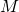) that indicates whether someone is male.

If this is the world we’re in, then when we set up our model we need to think about what question we want to answer. If we decide to model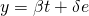thenis the effect of testosterone on muscle mass, andis the effect of exercise (3).

If we decide to model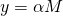then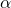is the average difference between men and women in terms of muscle mass.

Things would get a bit odd if we did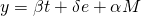.

Unless ‘being male’ has an effect on muscle mass that doesn’t work through testosterone levels, then the usual value ofproduced by this model should be equal to 0 (4). Being a man has no effect on muscle mass, controlling for testosterone. This is entirely true, and also almost completely useless if what we wanted to know was what effect being a man has on muscle mass. This is an Everest regression.

If you’re reading a paper that finds no effect of X controlling for Z, it’s worth thinking about whether the control is sensible. If it’s an essential characteristic of X, then the results might not be useful.

1. Citation needed
2. Don’t start.
3. Grossly simplified, not allowing for interactions, not checking the functional form, omitting thousands of variables, etc. It’s a toy model!
4. Loosely, because I don’t want to fiddle around with LaTeX too much and I can’t be bothered doing error terms:

Split the regressors for the first equation into two groups,and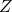. Label their respective vectors of coefficientsand. Setas the vector of outcomes.

We also have thatis determined in part by, withthe vector of regression coefficients.

Then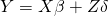and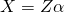In the first model we have. If we omitthen we getWhich then gives usAnd the end result is that we estimate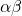, which is roughly the effect of Z on X, and of that change in X on Y.